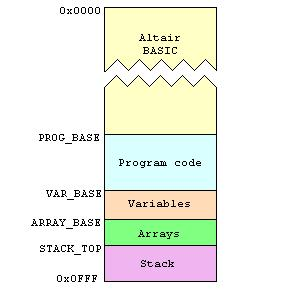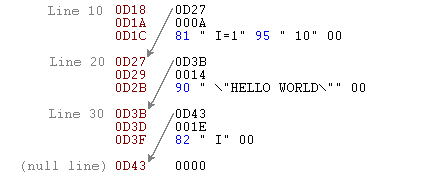The BASIC Interpreter - Explained

blah

How Basic arranged memory

Here's how Basic arranged the 4K of memory available to it. Most of memory was of course occupied by BASIC itself - from 76% to 82% depending on what optional inline functions had been selected during initialisation - with the remaining few hundred bytes at the top for the program code, space for variables, and of course stack space.Lets consider the blocks of memory that follow Basic's own code in turn :

The minimum amount of stack space is 18 bytes - at initialisation, after the user has stated the options they want, the amount of space is reported as "X BYTES FREE", where X is 4096 minus (amount needed for Basic, plus 18 bytes for the stack). With all optional inline functions selected - SIN, RND, and SQR - X works out to 727 bytes. With no optional inline functions selected, the amount increases to 973 bytes.

Program Code

For efficiency, each line of the program would be 'tokenised' before being stored in program space. This tokenisation involved the simple replacement of keywords with keyword IDs. These keyword IDs occupied a single byte, and were easily distinguished from other bytes of the program since they had their top bit set - ie they were in the range of 0x80 to 0xFF.

Consider this line of input :

FOR I=1 TO 10

This would be tokenised to :

81 " I=1" 95 " 10"

Which is 0x81 (keyword ID for 'FOR') followed by the string " I=1", followed by 0x95 (keyword ID for 'TO') followed by the string " 10". This is 9 bytes, compared to 13 bytes for the untokenised input.

This particular example line of input is meaningless unless it is part of a larger program. As you should know already, each line of a program is prefixed with a line number. These line numbers get stored as 16-bit integers preceding the tokenised line content. Additionally, each line is stored with a pointer to the following line. Let's consider the example input again, this time as a part of a larger program :

10 FOR I=1 TO 10
20 PRINT "HELLO WORLD"
30 NEXT I

Assuming that the beginning of program memory was at 0D18, this program would be stored in memory like this:So as you can see, each program line has three components :

• Pointer to the next line
• Line number
• Tokenised line content

The final line of the program - the last one in the above diagram - is always present and is always a null pointer to the non-existent next line. This null line, just two bytes long, is there to mark the end of the program.

Variables

The variable support in this version of Basic is rather limited. There only permitted type of variables is numeric - no strings, structs, and of course no distinction between integers and floating-point numbers. All variables are stored and treated as floating-point.

The second restriction is that variable names were a maximum of two characters in length : the first (mandatory) character had to be alphabetic, and the second (optional) character had to be a digit. Thus the following declarations are invalid :

 LET FOO=1 cross LET A="HELLO" cross LET AB=A cross

Whereas these declarations are valid :

 LET A=1 tick LET B=2.5 tick LET B2=5.6 tick

The fixed-length of variable names greatly simplified their storage. Each variable occupies 6 bytes : two bytes for the name, and four bytes for the floating-point value (fixme: link to fp).

Arrays

Arrays are stored seperately in their own block which immediately follows normal variables and is pointed to by VAR_ARRAY_BASE. An array is declared with the DIM keyword, and this version of Basic has the curious property where declaring an array of n elements results in n+1 elements being allocated, addressable with subscript values from 0 to n inclusive. Thus the following is quite legal :

DIM A(2)
A(0) = 1
A(1) = 2
A(2) = 3

but :

A(3) = 4

results in a Bad Subscript (BS) error.

An array is stored similarly to normal variables in that we lead with the two-byte variable name. This is followed by a 16-bit integer denoting the size in bytes of the array elements; and finally the array elements themselves (4 bytes each). The example array A(2) shown above, if stored at address 0D20, would appear like this :

 Address Bytes Value Description 0D20 0x4100 'A\0' Variable name 0D22 0x000C Total size, in bytes, of the array elements. 0D24 0x81000000 1 Element 0 value 0D28 0x82000000 2 Element 1 value 0D2C 0x82400000 3 Element 2 value

Program Flow

When a program is RUN, execution begins on the first line of the program. When a line has finished, execution passes to the next line and so on, until the end of the program or a END or STOP instruction is reached.

This is too simple for all but the simplest programs - there are two mechanisms in Basic for altering program flow so that code can run in loops and subroutines be called. These mechanisms are FOR/NEXT for looping, and GOSUB/RETURN for subroutines.

In both FOR and GOSUB cases, the stack is used to store specific information about the program line to return to.